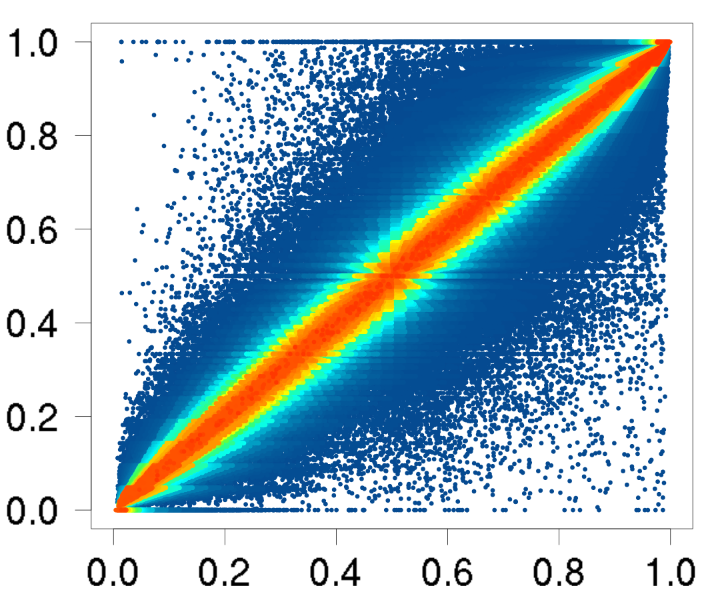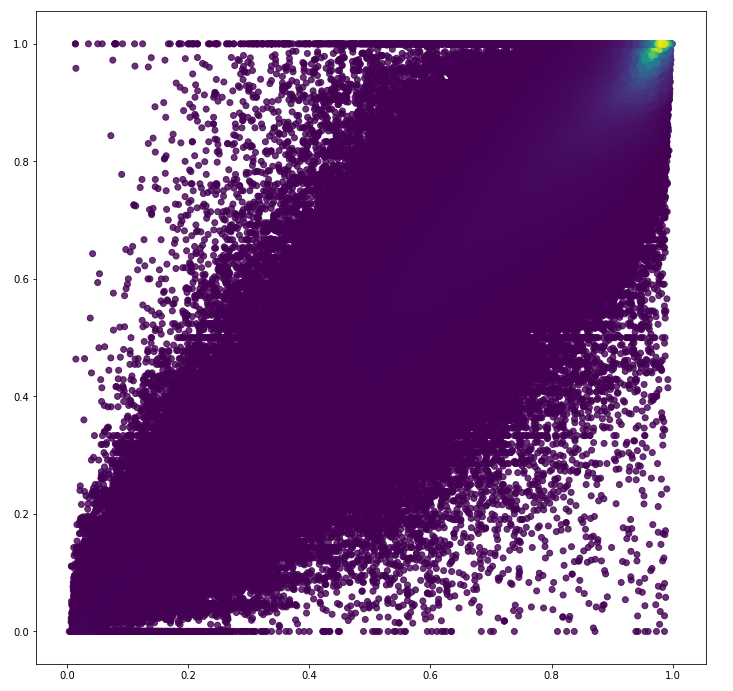Instantly share code, notes, and snippets.

#joferkington/fast_kde.py Last active Aug 8, 2019

 import numpy as np import scipy.sparse import scipy.ndimage import scipy.stats import scipy.signal import matplotlib.pyplot as plt def main(): x, y = generate_data(int(1e7)) grid, extents, density = fast_kde(x, y, sample=True) image_example(grid, extents) scatter_example(x, y, density) plt.show() def generate_data(num): x = 10 * np.random.random(num) y = x**2 + np.random.normal(0, 5, num)**2 return x, y def image_example(grid, extents): fig, ax = plt.subplots() im = ax.imshow(grid, origin='lower', extent=extents, aspect='auto', cmap='gist_earth_r') fig.colorbar(im) def scatter_example(x, y, density, num_points=10000): # Randomly draw a subset based on the _inverse_ of the estimated density prob = 1.0 / density prob /= prob.sum() subset = np.random.choice(np.arange(x.size), num_points, False, prob) x, y, density = x[subset], y[subset], density[subset] fig, ax = plt.subplots() ax.scatter(x, y, c=density, cmap='gist_earth_r') ax.axis('tight') def fast_kde(x, y, gridsize=(400, 400), extents=None, weights=None, sample=False): """ Performs a gaussian kernel density estimate over a regular grid using a convolution of the gaussian kernel with a 2D histogram of the data. This function is typically several orders of magnitude faster than scipy.stats.kde.gaussian_kde for large (>1e7) numbers of points and produces an essentially identical result. Input: x: array-like The x-coords of the input data points y: array-like The y-coords of the input data points gridsize: tuple, optional An (nx,ny) tuple of the size of the output grid. Defaults to (400, 400). extents: tuple, optional A (xmin, xmax, ymin, ymax) tuple of the extents of output grid. Defaults to min/max of x & y input. weights: array-like or None, optional An array of the same shape as x & y that weighs each sample (x_i, y_i) by each value in weights (w_i). Defaults to an array of ones the same size as x & y. sample: boolean Whether or not to return the estimated density at each location. Defaults to False Output: density : 2D array of shape *gridsize* The estimated probability distribution function on a regular grid extents : tuple xmin, xmax, ymin, ymax sampled_density : 1D array of len(*x*) Only returned if *sample* is True. The estimated density at each point. """ #---- Setup -------------------------------------------------------------- x, y = np.atleast_1d([x, y]) x, y = x.reshape(-1), y.reshape(-1) if x.size != y.size: raise ValueError('Input x & y arrays must be the same size!') nx, ny = gridsize n = x.size if weights is None: # Default: Weight all points equally weights = np.ones(n) else: weights = np.squeeze(np.asarray(weights)) if weights.size != x.size: raise ValueError('Input weights must be an array of the same size' ' as input x & y arrays!') # Default extents are the extent of the data if extents is None: xmin, xmax = x.min(), x.max() ymin, ymax = y.min(), y.max() else: xmin, xmax, ymin, ymax = map(float, extents) extents = xmin, xmax, ymin, ymax dx = (xmax - xmin) / (nx - 1) dy = (ymax - ymin) / (ny - 1) #---- Preliminary Calculations ------------------------------------------- # Most of this is a hack to re-implment np.histogram2d using `coo_matrix` # for better memory/speed performance with huge numbers of points. # First convert x & y over to pixel coordinates # (Avoiding np.digitize due to excessive memory usage!) ij = np.column_stack((y, x)) ij -= [ymin, xmin] ij /= [dy, dx] ij = np.floor(ij, ij).T # Next, make a 2D histogram of x & y # Avoiding np.histogram2d due to excessive memory usage with many points grid = scipy.sparse.coo_matrix((weights, ij), shape=(ny, nx)).toarray() # Calculate the covariance matrix (in pixel coords) cov = image_cov(grid) # Scaling factor for bandwidth scotts_factor = np.power(n, -1.0 / 6) # For 2D #---- Make the gaussian kernel ------------------------------------------- # First, determine how big the kernel needs to be std_devs = np.diag(np.sqrt(cov)) kern_nx, kern_ny = np.round(scotts_factor * 2 * np.pi * std_devs) kern_nx, kern_ny = int(kern_nx), int(kern_ny) # Determine the bandwidth to use for the gaussian kernel inv_cov = np.linalg.inv(cov * scotts_factor**2) # x & y (pixel) coords of the kernel grid, with = <0,0> in center xx = np.arange(kern_nx, dtype=np.float) - kern_nx / 2.0 yy = np.arange(kern_ny, dtype=np.float) - kern_ny / 2.0 xx, yy = np.meshgrid(xx, yy) # Then evaluate the gaussian function on the kernel grid kernel = np.vstack((xx.flatten(), yy.flatten())) kernel = np.dot(inv_cov, kernel) * kernel kernel = np.sum(kernel, axis=0) / 2.0 kernel = np.exp(-kernel) kernel = kernel.reshape((kern_ny, kern_nx)) #---- Produce the kernel density estimate -------------------------------- # Convolve the gaussian kernel with the 2D histogram, producing a gaussian # kernel density estimate on a regular grid # Big kernel, use fft... if kern_nx * kern_ny > np.product(gridsize) / 4.0: grid = scipy.signal.fftconvolve(grid, kernel, mode='same') # Small kernel, use ndimage else: grid = scipy.ndimage.convolve(grid, kernel, mode='constant', cval=0) # Normalization factor to divide result by so that units are in the same # units as scipy.stats.kde.gaussian_kde's output. norm_factor = 2 * np.pi * cov * scotts_factor**2 norm_factor = np.linalg.det(norm_factor) norm_factor = n * dx * dy * np.sqrt(norm_factor) # Normalize the result grid /= norm_factor if sample: i, j = ij.astype(int) return grid, extents, grid[i, j] else: return grid, extents def image_cov(data): """Efficiently calculate the cov matrix of an image.""" def raw_moment(data, ix, iy, iord, jord): data = data * ix**iord * iy**jord return data.sum() ni, nj = data.shape iy, ix = np.mgrid[:ni, :nj] data_sum = data.sum() m10 = raw_moment(data, ix, iy, 1, 0) m01 = raw_moment(data, ix, iy, 0, 1) x_bar = m10 / data_sum y_bar = m01 / data_sum u11 = (raw_moment(data, ix, iy, 1, 1) - x_bar * m01) / data_sum u20 = (raw_moment(data, ix, iy, 2, 0) - x_bar * m10) / data_sum u02 = (raw_moment(data, ix, iy, 0, 2) - y_bar * m01) / data_sum cov = np.array([[u20, u11], [u11, u02]]) return cov if __name__ == '__main__': main()

### MikeDacre commented Apr 6, 2018

 This is amazing! Thanks for sharing. Do you have an idea of how to make this code show a more smooth plot even when the vast majority of the points are in a small area? Specifically, I have some R code that uses densCols ((cisVar)[https://github.com/TheFraserLab/cisVar/blob/31e5c8c92643c6ccc7efd0ac6d5b50e1cef252c5/scripts/plot_fun.R]) to plot some frequency data and produces this:Whereas when I use this fast kde method, I end up with this:I would really like to be able to adapt this code to do more general density plots even in highly asymmetric data but I am not sure how to do that. Maybe by adjusting the weights or the cmap?
Owner Author

### joferkington commented Apr 13, 2018

 @MikeDacre - Sorry I didn't notice your comment earlier! In that case, there's the correct way and then there's a shortcut. The shortcut is to just plot the log of the values. If you're not displaying a colorbar, that's the route you'll want to take. For example, plot `ax.scatter(x, y, c=np.log(density), cmap='gist_earth_r')` and you should get something that looks similar to the R plot. If you want to display a colorbar alongside it and still have the correct values, you'd want to change the `Normalize` type used for the color plotting. Something like `ax.scatter(x, y, c=density, cmap='gist_earth_r', norm=LogNorm(density.min(), density.max()))`.
to join this conversation on GitHub. Already have an account? Sign in to comment
You can’t perform that action at this time.